Latest Banking jobs   »   Quantitative Aptitude Quiz For SBI Clerk...

# Quantitative Aptitude Quiz For SBI Clerk Prelims 2022- 22nd May

Directions (1-4): Each of the following questions below consists of a question and two statements numbered I and II given. You have to decide whether the data provided in the statements is sufficient to answer the questions.
Q1. Find the cost price of article by shopkeeper on selling the article at Rs. 240 ?
If the article sold at 25% more the profit earned will be Rs. 40.
(II) Marked price of article is Rs. 400 and profit% is equal to discount% and profit% is 40%.

(a) if the data given in statement I alone is sufficient to answer the question while the data in statement II alone is not sufficient to answer the question.
(b) if the data given in statement II alone is sufficient to answer the question while the data in statement I alone is not sufficient to answer the question.
(c) if the data either in statement I alone or in statement II alone is sufficient to answer the question.
(d) If the data in neither statement I nor II is sufficient to answer the question.
(e) If the data in both statements I and II together is necessary to answer the question.

Q2. Find the volume of right circular cone ?
(I) Height of cone is 100% more than radius of cone.
(II) Area of base of cone is 154 cm².

(a) if the data given in statement I alone is sufficient to answer the question while the data in statement II alone is not sufficient to answer the question.
(b) if the data given in statement II alone is sufficient to answer the question while the data in statement I alone is not sufficient to answer the question.
(c) if the data either in statement I alone or in statement II alone is sufficient to answer the question.
(d) If the data in neither statement I nor II is sufficient to answer the question.
(e) If the data in both statements I and II together is necessary to answer the question.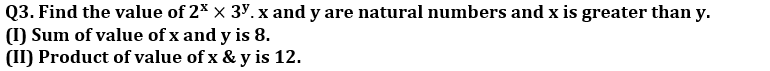(a) if the data given in statement I alone is sufficient to answer the question while the data in statement II alone is not sufficient to answer the question.
(b) if the data given in statement II alone is sufficient to answer the question while the data in statement I alone is not sufficient to answer the question.
(c) if the data either in statement I alone or in statement II alone is sufficient to answer the question.
(d) If the data in neither statement I nor II is sufficient to answer the question.
(e) If the data in both statements I and II together is necessary to answer the question.

Q4. Find the speed of boat in still water?
(I) Time taken by boat to cover 64 km in downstream is half the time taken by same boat to cover same distance in still water.
(II) Speed of stream is 5 km/hr

(a) if the data given in statement I alone is sufficient to answer the question while the data in statement II alone is not sufficient to answer the question.
(b) if the data given in statement II alone is sufficient to answer the question while the data in statement I alone is not sufficient to answer the question.
(c) if the data either in statement I alone or in statement II alone is sufficient to answer the question.
(d) If the data in neither statement I nor II is sufficient to answer the question.
(e) If the data in both statements I and II together is necessary to answer the question.

Q5. Hemant, Manoj and Mohit invested in partnership. Hemant invest Rs. 8000 for 5 months, Manoj invest Rs. 6000 for 3 months and Mohit invest Rs. 12000 for 2 months. Mohit is working partner so he will get 5% extra of total profit. Find profit share of Mohit if total profit at the end of the year is Rs.8200.
Rs.2380
Rs.2690
Rs.2740
Rs.2800
Rs.3800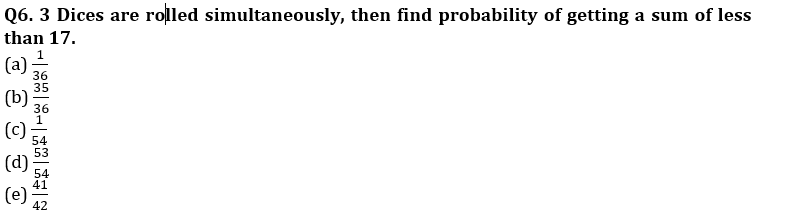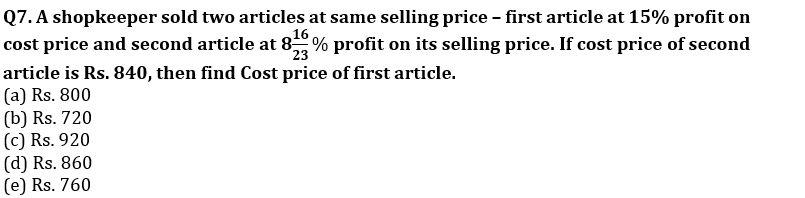Q8. On a sum of Rs. 6875, find difference between second year and third year C.I if rate of interest is 20% per Annum?
(a) Rs. 275
(b) Rs. 300
(c) Rs. 330
(d) Rs. 325
(e) Rs. 290

Q9. Area of base of a cylinder is 132cm² and height is half of square of radius of it’s base. Find volume of the cylinders?
(a) 2772 cm³
(b) 3113 cm³
(c) 2376 cm³
(d) 924 cm³
(e) 1452 cm³

Q10. A and B lent equal amount of money at simple interest at the rate of 6% and 5% per annum at the same time. A recovered his amount 8 months earlier than B and the amount recovered in each case is Rs 3240. What is the sum?
Rs 2400
Rs 3540
Rs 2850
Rs 2700
Rs 5400

Directions (11-15): Bar graph given below shows percentage of students out of total selected students who written UPSC exam in English language in five different years. Read the data carefully and answer the questions.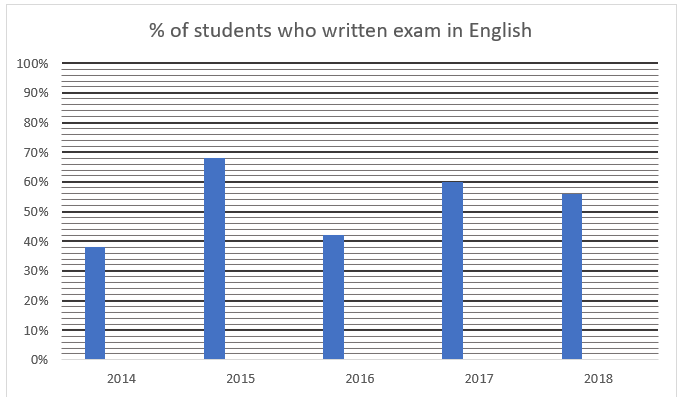Note: – Exam can be written only in two languages either English or Hindi.

Q11. If no. of students who written exam in Hind in year 2017 are 450 less than no. of students who written exam in English in the same year and total no. of selection in 2017 are 10% less than total no. of selection in 2015, then find total selection in 2015?
(a) 2250
(b) 2325
(c) 2400
(d) 2500
(e) 2025

Q12. Ratio of total selection in year 2014 to that of 2016 is 2:3. Find ratio of students who written exam in Hindi and got selected in 2014 to that of in 2016?
(a) 62:87
(b) 31:43
(c) 11:19
(d) 7:11
(e) 62:89

Q13. If total no. of selection in each the given year are same and average no. of students who written exam in English and got selected in 2014, 2015 and 2018 are 540. Find no. of selection in each year?
(a) 1200
(b) 1000
(c) 1500
(d) 800
(e) 900

Q14. If no. students who written exam in Hindi and got selected in 2015 and 2018 are equal, find ratio of total selection in 2015 to 2018?
(a) 5:4
(b) 7:8
(c) 17:14
(d) 11:8
(e) 17:8

Q15. If in 2014 and 2017 no. of students who written exam in English and got selected are 380 and 480 respectively, find total no. of selection in 2017 are how much percent more/less than that of 2014?
(a) 20% less
(b) 25% more
(c) 20% more
(d) 25% less
(e) 15% more

Solutions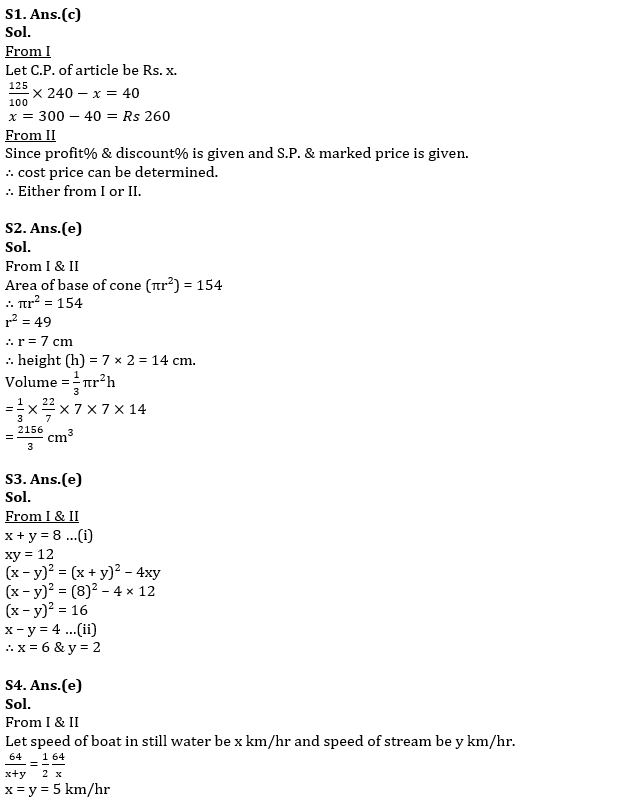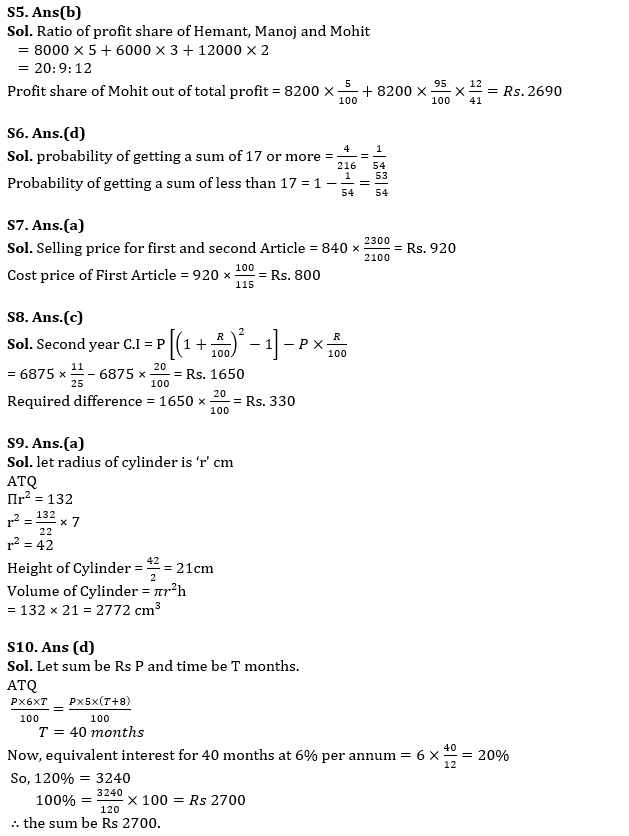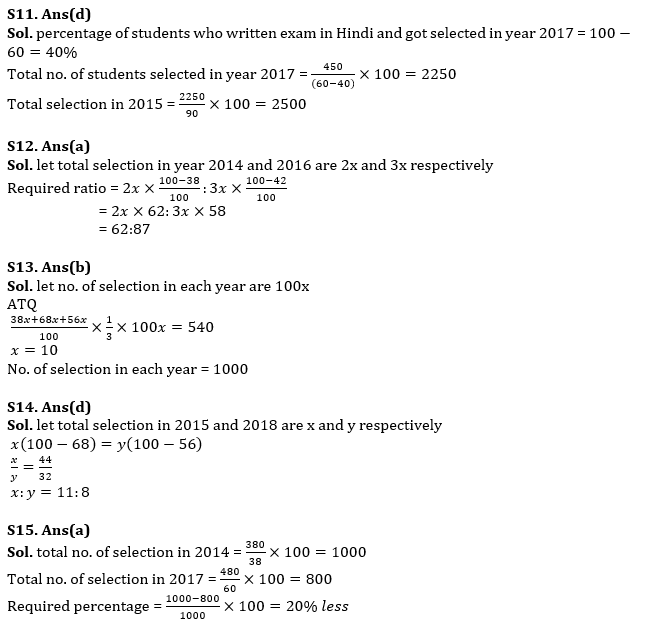#### Congratulations!Union Budget 2023-24: Free PDF